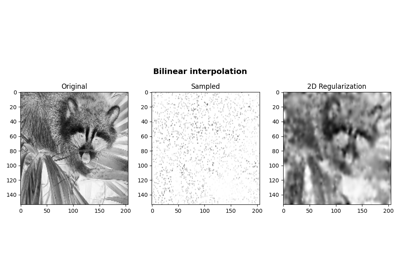pylops.signalprocessing.Bilinear¶

class pylops.signalprocessing.Bilinear(iava, dims, dtype='float64')[source]

Bilinear interpolation operator.

Apply bilinear interpolation onto fractionary positions iava along the first two axes of a n-dimensional array.

Note

The vector iava should contain unique pais. If the same pair is repeated twice an error will be raised.

Parameters: iava : Array of size $$[2 \times n_{ava}]$$ containing pairs of floating indices of locations of available samples for interpolation. dims : list Number of samples for each dimension dtype : str, optional Type of elements in input array. ValueError If the vector iava contains repeated values.

Notes

Bilinear interpolation of a subset of $$N$$ values at locations iava from an input n-dimensional vector $$\mathbf{x}$$ of size $$[m_1 \times m_2 \times ... \times m_{ndim}]$$ can be expressed as:

$y_{\mathbf{i}} = (1-w^0_{i}) (1-w^1_{i}) x_{l^{l,0}_i, l^{l,1}_i} + w^0_{i} (1-w^1_{i}) x_{l^{r,0}_i, l^{l,1}_i} + (1-w^0_{i}) w^1_{i} x_{l^{l,0}_i, l^{r,1}_i} + w^0_{i} w^1_{i} x_{l^{r,0}_i, l^{r,1}_i} \quad \forall i=1,2,...,M$

where $$\mathbf{l^{l,0}}=[\lfloor l_1^0 \rfloor, \lfloor l_2^0 \rfloor, ..., \lfloor l_N^0 \rfloor]$$, $$\mathbf{l^{l,1}}=[\lfloor l_1^1 \rfloor, \lfloor l_2^1 \rfloor, ..., \lfloor l_N^1 \rfloor]$$, $$\mathbf{l^{r,0}}=[\lfloor l_1^0 \rfloor + 1, \lfloor l_2^0 \rfloor + 1, ..., \lfloor l_N^0 \rfloor + 1]$$, $$\mathbf{l^{r,1}}=[\lfloor l_1^1 \rfloor + 1, \lfloor l_2^1 \rfloor + 1, ..., \lfloor l_N^1 \rfloor + 1]$$, are vectors containing the indices of the original array at which samples are taken, and $$\mathbf{w^j}=[l_1^i - \lfloor l_1^i \rfloor, l_2^i - \lfloor l_2^i \rfloor, ..., l_N^i - \lfloor l_N^i \rfloor]$$ ($$\forall j=0,1$$) are the bilinear interpolation weights.

Attributes: shape : tuple Operator shape explicit : bool Operator contains a matrix that can be solved explicitly (True) or not (False)

Methods

 __init__(iava, dims[, dtype]) Initialize this LinearOperator. adjoint() Hermitian adjoint. apply_columns(cols) Apply subset of columns of operator cond([uselobpcg]) Condition number of linear operator. conj() Complex conjugate operator div(y[, niter]) Solve the linear problem $$\mathbf{y}=\mathbf{A}\mathbf{x}$$. dot(x) Matrix-matrix or matrix-vector multiplication. eigs([neigs, symmetric, niter, uselobpcg]) Most significant eigenvalues of linear operator. matmat(X) Matrix-matrix multiplication. matvec(x) Matrix-vector multiplication. rmatmat(X) Matrix-matrix multiplication. rmatvec(x) Adjoint matrix-vector multiplication. todense([backend]) Return dense matrix. toimag([forw, adj]) Imag operator toreal([forw, adj]) Real operator tosparse() Return sparse matrix. trace([neval, method, backend]) Trace of linear operator. transpose() Transpose this linear operator.

Examples using pylops.signalprocessing.Bilinear¶Bilinear Interpolation